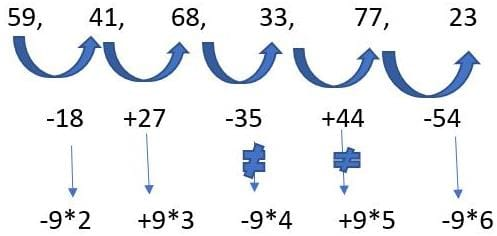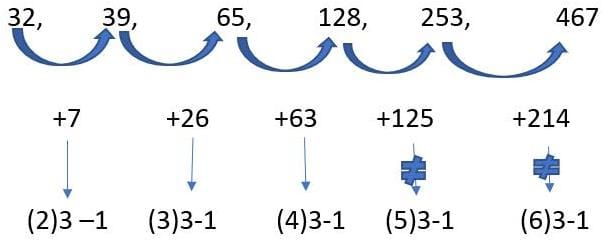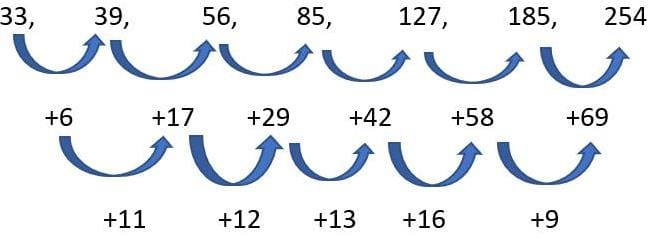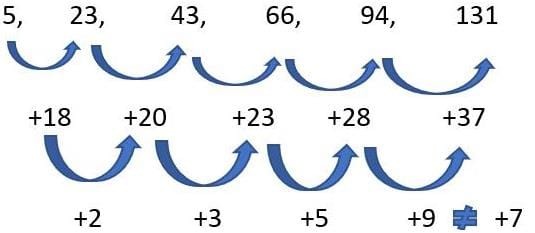CAT  >  Practice Test: Wrong Number Series

# Practice Test: Wrong Number Series

Test Description

## 10 Questions MCQ Test Logical Reasoning (LR) and Data Interpretation (DI) | Practice Test: Wrong Number Series

Practice Test: Wrong Number Series for CAT 2022 is part of Logical Reasoning (LR) and Data Interpretation (DI) preparation. The Practice Test: Wrong Number Series questions and answers have been prepared according to the CAT exam syllabus.The Practice Test: Wrong Number Series MCQs are made for CAT 2022 Exam. Find important definitions, questions, notes, meanings, examples, exercises, MCQs and online tests for Practice Test: Wrong Number Series below.
Solutions of Practice Test: Wrong Number Series questions in English are available as part of our Logical Reasoning (LR) and Data Interpretation (DI) for CAT & Practice Test: Wrong Number Series solutions in Hindi for Logical Reasoning (LR) and Data Interpretation (DI) course. Download more important topics, notes, lectures and mock test series for CAT Exam by signing up for free. Attempt Practice Test: Wrong Number Series | 10 questions in 20 minutes | Mock test for CAT preparation | Free important questions MCQ to study Logical Reasoning (LR) and Data Interpretation (DI) for CAT Exam | Download free PDF with solutions
 1 Crore+ students have signed up on EduRev. Have you?
Practice Test: Wrong Number Series - Question 1

### Wrong Number Series: 59, 41, 68, 33, 77, 23

Detailed Solution for Practice Test: Wrong Number Series - Question 1Therefore, wrong no. is 33

Practice Test: Wrong Number Series - Question 2

### Wrong Number Series: 9, 5, 6, 10.5, 23, 90, 183

Detailed Solution for Practice Test: Wrong Number Series - Question 2

The solution is :
9 * .5 + .5 = 5
5 * 1 + 1 = 6
6 * 1.5 + 1.5 = 10.5
10.5 * 2 + 2 = 23
23 * 2.5 + 2.5 = 60 ≠ 90
60 * 3 + 3 = 183
Therefore, wrong no. is 90

Practice Test: Wrong Number Series - Question 3

### Wrong Number Series: 318, 168, 78, 38, 18, 8, 3

Detailed Solution for Practice Test: Wrong Number Series - Question 3

The solution is :
8/2 – 1 = 3
18/2 - 1 = 8
38/2 – 1 = 18
78/2 – 1 = 38
168/2 – 1 = 83 ≠ 78
318/2 -1 = 158  ≠ 168
Therefore, wrong no. is 168

Practice Test: Wrong Number Series - Question 4

Wrong Number Series: 3, 8, 18, 38, 80, 158, 318

Detailed Solution for Practice Test: Wrong Number Series - Question 4

The solution is:
3 * 2 + 2 = 8
8 * 2 + 2 = 18
18 * 2 + 2 = 38
38 * 2 + 2 = 78 ≠ 80
78 * 2 + 2 = 158
158 * 2 + 2 = 318
Therefore, wrong no. is 80

Practice Test: Wrong Number Series - Question 5

Wrong Number Series: 120, 143, 168, 195, 226, 255

Detailed Solution for Practice Test: Wrong Number Series - Question 5

(11)2 – 1 = 120
(12)2 – 1 = 143
(13)2 – 1 = 168
(14)2 – 1 = 195
(15)2 – 1 = 224 ≠ 226
(16)2 – 1 = 255

Practice Test: Wrong Number Series - Question 6

Wrong Number Series: 32, 39, 65, 128, 253, 467

Detailed Solution for Practice Test: Wrong Number Series - Question 6Therefore, wrong no. is 253

Practice Test: Wrong Number Series - Question 7

Wrong Number Series: 33, 39, 56, 85, 127, 185, 254

Detailed Solution for Practice Test: Wrong Number Series - Question 7Therefore, it must be 42+14 = 56   i.e.
127 + 56 = 183
56 + 15 = 71  i.e. 183 + 71 = 254
Hence, wrong no. is 185

Practice Test: Wrong Number Series - Question 8

Wrong Number Series: 7, 9, 21, 67, 275, 1371, 8233

Detailed Solution for Practice Test: Wrong Number Series - Question 8

The solution is :
7 * 1 + 2 = 9
9 * 2 + 3 = 21
21 * 3 + 4 = 67
67 * 4 + 5 = 273  ≠  275
273 * 5 + 6 = 1371
1371 * 6 + 7 = 8233
Therefore, wrong no. is 275

Practice Test: Wrong Number Series - Question 9

Wrong Number Series: 3, 6, 15, 45, 157, 630, 2835

Detailed Solution for Practice Test: Wrong Number Series - Question 9

The solution is :
3 * 2 = 6
6 * 2.5 = 15
15 * 3 = 45
45 * 3.5 = 157.5 ≠ 157
157.5 * 4 = 630
630 * 4.5 = 2835
Therefore, wrong no. is 157

Practice Test: Wrong Number Series - Question 10

Wrong Number Series: 5, 23, 43, 66, 94, 131

Detailed Solution for Practice Test: Wrong Number Series - Question 10Because, difference of the difference is prime numbers in increasing order therefore, wrong no. is 131

## Logical Reasoning (LR) and Data Interpretation (DI)

97 videos|83 docs|119 tests
 Use Code STAYHOME200 and get INR 200 additional OFF Use Coupon Code
Information about Practice Test: Wrong Number Series Page
In this test you can find the Exam questions for Practice Test: Wrong Number Series solved & explained in the simplest way possible. Besides giving Questions and answers for Practice Test: Wrong Number Series, EduRev gives you an ample number of Online tests for practice

## Logical Reasoning (LR) and Data Interpretation (DI)

97 videos|83 docs|119 tests

### How to Prepare for CAT

Read our guide to prepare for CAT which is created by Toppers & the best Teachers